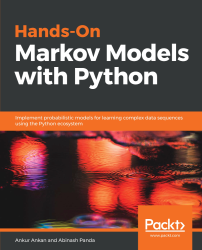•#### Hands-On Markov Models with Python#### Overview of this book

Hidden Markov Model (HMM) is a statistical model based on the Markov chain concept. Hands-On Markov Models with Python helps you get to grips with HMMs and different inference algorithms by working on real-world problems. The hands-on examples explored in the book help you simplify the process flow in machine learning by using Markov model concepts, thereby making it accessible to everyone. Once you’ve covered the basic concepts of Markov chains, you’ll get insights into Markov processes, models, and types with the help of practical examples. After grasping these fundamentals, you’ll move on to learning about the different algorithms used in inferences and applying them in state and parameter inference. In addition to this, you’ll explore the Bayesian approach of inference and learn how to apply it in HMMs. In further chapters, you’ll discover how to use HMMs in time series analysis and natural language processing (NLP) using Python. You’ll also learn to apply HMM to image processing using 2D-HMM to segment images. Finally, you’ll understand how to apply HMM for reinforcement learning (RL) with the help of Q-Learning, and use this technique for single-stock and multi-stock algorithmic trading. By the end of this book, you will have grasped how to build your own Markov and hidden Markov models on complex datasets in order to apply them to projects.
PrefaceFree Chapter
Introduction to the Markov ProcessHidden Markov ModelsState Inference - Predicting the StatesParameter Learning Using Maximum LikelihoodParameter Inference Using the Bayesian ApproachTime Series PredictingNatural Language Processing2D HMM for Image ProcessingMarkov Decision ProcessOther Books You May Enjoy# Recap of 1D HMM

Let's recap how 1D HMMs work, which we discussed in the previous chapters of this book. We have seen that HMM is a just a process over Markov chains. At any point in time, an HMM is in one of the possible states, and the next state that the model will transition to depends on the current state and the transition probability of the model.

Suppose that there are M = {1, 2, ..., M} possible states for HMM, and the transition probability of going from some state i to state j is given by ai,j. For such a model, if at time t-1 the model is at state i, then at time t it would be in state j with a probability of ai,j. This probability is known as the transition probability. Also, we have defined the observed variable in the model, which only depends on the current state of our hidden variable. We can define the observed variable at time t as ut, so let's say...